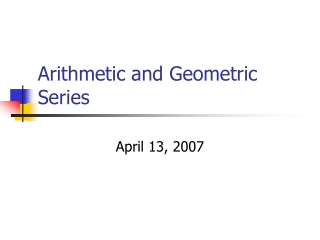DownloadDownload PresentationArithmetic and Geometric Series

# Arithmetic and Geometric Series

Télécharger la présentation## Arithmetic and Geometric Series

- - - - - - - - - - - - - - - - - - - - - - - - - - - E N D - - - - - - - - - - - - - - - - - - - - - - - - - - -
##### Presentation Transcript

1. Arithmetic and Geometric Series April 13, 2007

2. Sequence vs. Series • Sequence – a set of numbers that follow a general rule (nth term formula) • Examples: 1, 8, 15, 22, 29, … -8, 2, -1/2, 1/8, … • Series – the sum of the terms in a sequence of numbers • Examples: 1 + 8 + 15 + 22 + 29 + … -8 + 2 – 1/2 + 1/8 - …

3. Finite vs. Infinite • a Finite series has an end • Example: -9 – 3 + 3 + 9 + 15 2 + 4 + 8 + … + 1024 • an Infinite series continues indefinitely • Example: 16 + 12 + 9 + …

4. Finite Arithmetic Series • WARNING: you MUST have an Arithmetic Series to use this formula • Reminder: the nth term formula for an arithmetic series is • an = a1 + (n – 1)d

5. Example Find the sum of the first 60 terms in the arithmetic series 9 + 14 + 19 + … n = 60 a1 = 9 a60 = ? a60 = a1 + (60 – 1)d = 9 + (60 – 1)5 = 304 S60 = 60 (9 + 304) 2 = 30 (313) = 9390

6. Finite Geometric Series • WARNING: you MUST have a Geometric Series to use this formula

7. Example Sn = a1 (1 – rn) 1 – r • Find the sum of the first ten terms of the geometric series 16 – 48 + 144 – 432 + … n = 10 a1 = 16 r = -3 S10 = 16 [1 – (-3)10] 1 – (-3) = 16 (1 – 59,049) 4 = -236,192

8. Infinite Geometric Series(condition |r|<1) • Finite Geometric Series • Sn = a1 (1 – rn) 1 – r if |r|<1, what happens to rn when n becomes very large (i.e. approaches infinity) rn 0 • Infinite Geometric Series • S = a1__ 1 – r

9. Example S = a1_ 1 – r • Find the sum of the series 21 – 3 + 3/7 - … Is |r| < 1? r = -1/7, so |r| < 1 S = 21____ 1 – (-1/7) S = 147 8 Fancy Math Language: Converges to 147/8

10. Example S = a1_ 1 – r • Find the sum of the series 1 + 3 + 9 + 27 + … Is |r| < 1? r = 3, so |r| > 1 the condition is not met So the sum does not exist Fancy Math Language: Diverges